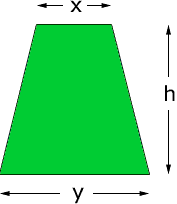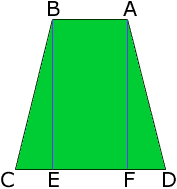Quandaries and Queries What is the total acreage of a pie-shaped piece of land that is: 146' front 85' back 246' each side The front and back lines are fairly straight....a trapezoidal shape. Thanks, Doak Hi Doak, The area of a trapezoid is the average of the lengths of the parallel sides, times the distance between these two sides.area = (x + y)/2h A development of this expression can be found in the answer to a previous problem. You sent us the lengths of the two parallel sides x = 85 feet y = 146 feet Since the lengths of the other two sides are equal, the symmetry in the diagram below can be used to show that triangles AFD and BCD are congruent.Thus |CE| = |FD| and thus 146 = |CD| = |CE| + |EF| + |FD| = |CE| + 85 + |FD| so |FD| = (146 - 85)/2 = 30.5 feet Triangle AFD is a right triangle so, using Pythagoras' Theorem h = |AF| = sqrt(|AD|2 - |FD|2) = sqrt(2462 - 30.52) = 244.1 feet This the area, in square feet is  (146 + 85)/2244.1 = 28,194.55 There are 23,560 square feet in an acre so the area, in acres is  28,194.55/23,560 = 1.2 Penny Go to Math Central